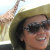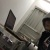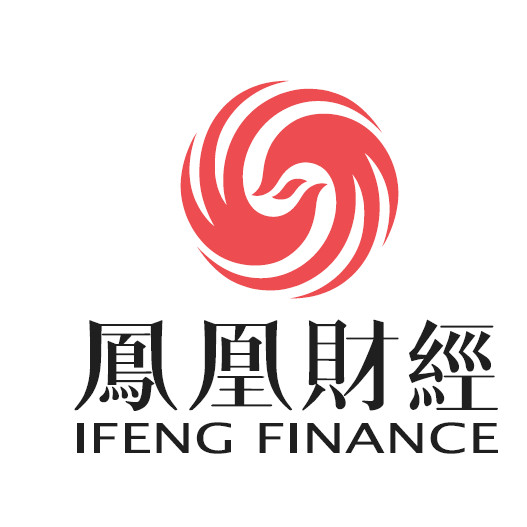International Joint Conference on Biometrics于2006年合并了AVBPA（基于音频和视频的个人认证）、ICBA（国际生物认证会议）和其他生物认证研讨会，已成为领先的生物认证国际会议。该会议的主题包括当前生物测定学研究和应用的所有领域。官网链接：http://www.icb2019.org/0+0+0+0+0+0+0+0+0+0+0+0+0+0+0+0+0+0+0+0+0+0+0+0+#财新数据通#【【研报精华】华为苹果销量超预期 消费电子供应链大年来了？】消费电子行业三季报业绩预计实现回暖，加上新机密集发布催化了市场情绪，近几个月电子行业显著跑赢大盘http://t.cn/Ai3H5icb（来自财新客户端）0+0+0+0+0+0+0+0+0+0+0+0+0+0+0+0+0+0+1+11+0+
Top